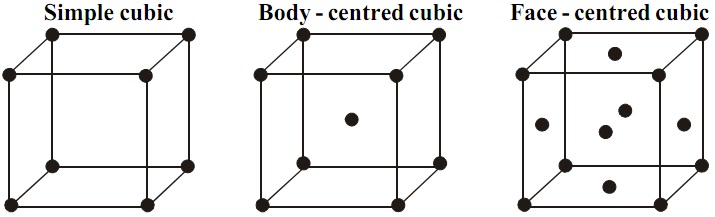# Cubic Unit Cells

Of the seven crystal systems, in the cubic crystal system the three repeat distances are equal and all the three angles are right angles.

### Number of atoms per unit cell

In unit cells the atoms can be on the corners, in the body center and on face centers. All the atoms do not belong to a single unit cell. These are shared among different unit cells.Simple Cubic Unit Cell

The simple or primitive unit cell has the atoms at the corners of the cube. A lattice point at the corner of the unit cell is shared by eight unit cells. Therefore, the contribution of an atom at the corner to the unit cell is 1/8.

Number of corner atoms = 8

Number of unit cells sharing atoms of the corner = 8

Number of atoms in a simple cubic unit cell = 8 × 1/8 = 1

Body Centered Cubic Unit Cell

A body centered cubic (bcc) unit cell has lattice points not only at the corners but also at the center of the cube. The atom in the center of the cube belongs entirely to the unit cell, i.e., it is not shared by other unit cells. The eight corner atoms, as in the case of simple cubic unit cell, are shared by eight unit cells.

Number of atoms in a body centered cubic unit cell = 1 + 1 = 2

Face Centered Cubic Unit Cell

A face centered cubic (fcc) unit cell has atoms not only at the corners but also at the center of each face. Thus, it has eight lattice points at the corners and six at the face centers.

Number of atoms at the face center = 6

Number of unit cells sharing a face centered lattice point = 2

Contribution of the face centered atoms to the unit cell = 6 × 1/2 = 3

Number of atoms points in a face centered cubic unit cell = 1 + 3 = 4